# Getting Started with MATLAB, Simulink, and ROS

Sebastian Castro is back to talk about the basics of connecting MATLAB and Simulink with the Robot Operating System (ROS).

NOTE: As of R2015a, Robotics System Toolbox equipped MATLAB and Simulink with an official interface to ROS. In R2019b, this functionality has been moved to the newer ROS Toolbox. This post and videos have been created before the R2019b release, and you may find some differences in syntax and product naming.

# Overview

Robot Operating System (or ROS) is a commonly used framework for designing complex robotic systems. It is popular for building distributed robot software systems, as well as for its integration with packages for simulation, visualization, robotics algorithms, and more. ROS has become increasingly popular in industry as well, especially in the development of autonomous vehicles.

The ROS interface provided by ROS Toolbox lets you:

• Connect to ROS from any operating system supported by MATLAB and Simulink
• Leverage built-in functionality in MathWorks toolboxes – for example, control systems, computer vision, machine learning, signal processing, and state machine design
• Automatically generate standalone C++ based ROS nodes from algorithms designed in MATLAB and Simulink

In summary, MATLAB and Simulink can coexist with your ROS based workflow via desktop prototyping, deployment of standalone ROS nodes, or both. If you are looking to design even one small component of your system in MATLAB and Simulink, keep reading!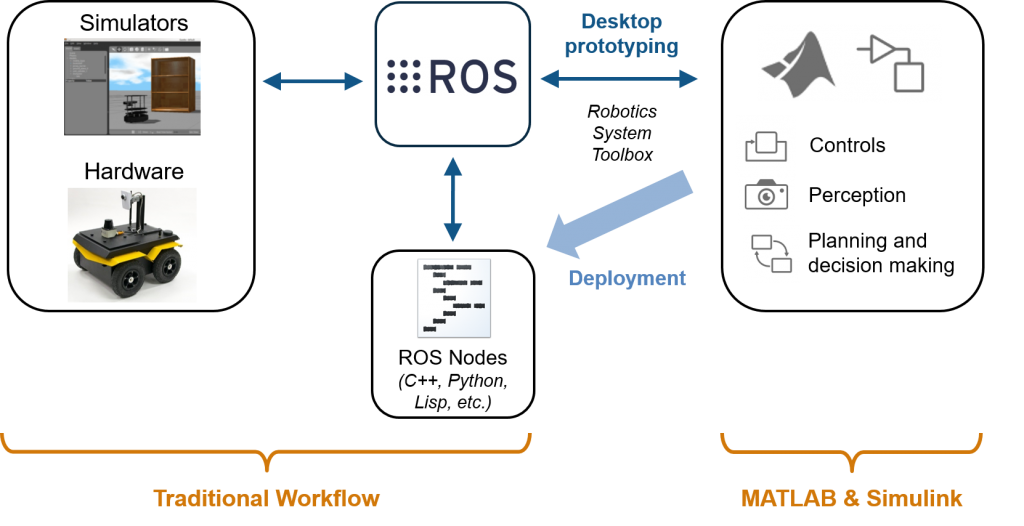# Connecting MATLAB and ROS

Robotics System Toolbox provides an interface for MATLAB scripting and desktop prototyping with ROS. This includes functionality for:

• Starting or connecting to a ROS master
• Working with topics (publish/subscribe), services, actions, and the parameter server
• Reading specialized messages (e.g., images, lidar scans, point clouds, and occupancy grids)

A simple MATLAB code snippet for a publish and subscribe based control algorithm looks as follows.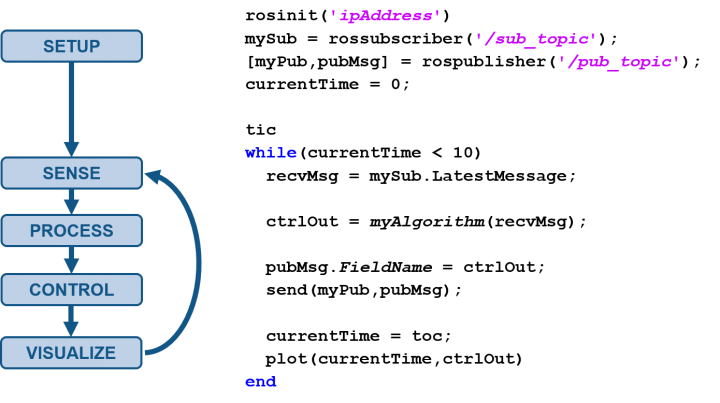In the example above, the algorithm is managed using a while-loop, which means the loop iterations are processed as quickly as possible. There are a few additional ways to schedule the execution of MATLAB code.

• Rates: You can use Rate and rosrate to slow down the control loop based on the CPU wall clock or the ROS master clock, respectively.
• Timers: Creating MATLAB timer objects lets you schedule function calls on different schedules without blocking. This means you can have multiple timers running in the background while still having access to the MATLAB command window.

NOTE: All the code running in timers and ROS subscriber callbacks will execute within a single thread, since the MATLAB environment is single-threaded to users (although several built-in functions use multithreading internally).

For more information on the MATLAB interface to ROS, watch the following video.
[VIDEO] MATLAB and Simulink Robotics Arena: Getting Started with MATLAB and ROS

ROS Toolbox also includes a Simulink block library for ROS connectivity. This includes functionality for:

• Working with topics (publish/subscribe) and the parameter server
• Representing ROS messages as Simulink bus signals for graphical programming
• Reading specialized messages (e.g., images and point clouds)

With Simulink, you can take advantage of block sample times and rate transitions to build multirate algorithms, as shown below.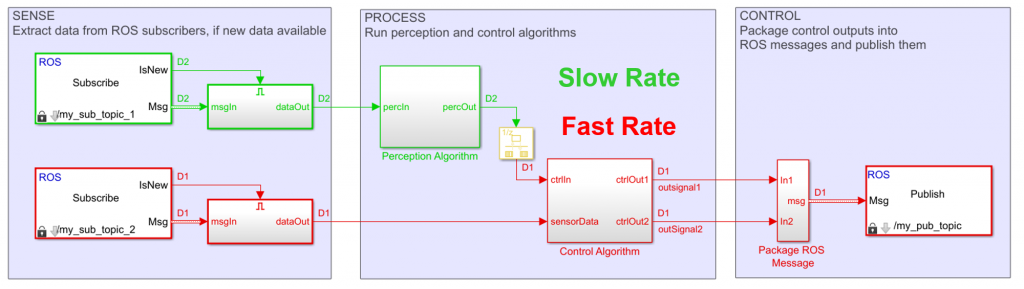Another benefit of the Simulink is the ability to combine MATLAB, Simulink, and Stateflow modeling language for different algorithm components.

• MATLAB code is good for components best expressed with textual programming, such as array/matrix operations and machine learning. If you have algorithms written in MATLAB, you can call them in Simulink using MATLAB Function blocks.
• Simulink blocks are good for control algorithms, which are usually expressed as block diagrams. You can also take advantage of built-in block libraries for signal processing, computer vision, robotics algorithms, and more.
• Stateflow charts are good for decision-based logic, state machines, tabular logic, and time-based or event-based scheduling.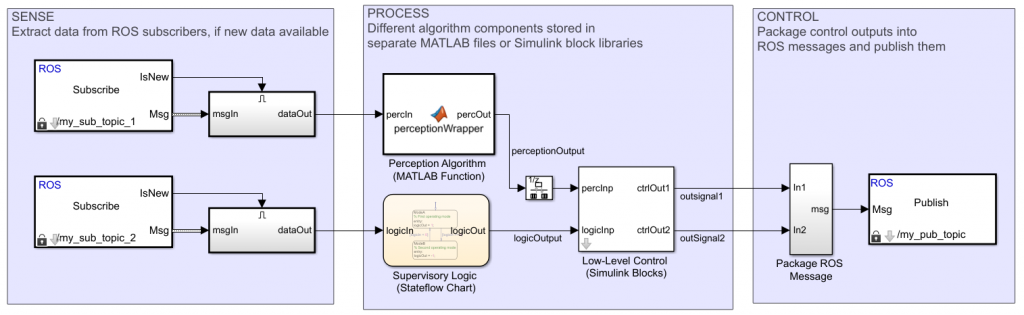[VIDEO] MATLAB and Simulink Robotics Arena: Getting Started with Simulink and ROS

# Generating Standalone ROS Nodes

We discussed how MATLAB and Simulink can help you design and verify robotics algorithms from your desktop. Even if your robot’s onboard computer meets the requirements to install and run MATLAB, you probably don’t need the overhead of these design and verification tools on the final system. On the other hand, installing MATLAB is completely out of the question for low-cost ROS enabled platforms such as Raspberry Pi.

As you transition to your final implementation, you could manually port the MATLAB and Simulink prototype algorithms to work on your robot. Alternatively, you could consider automating this process with code generation. This can speed up the development process and remove manual implementation errors.

The two code generation approaches are:

• Generate reusable code: Use MATLAB Coder, Simulink Coder, and Embedded Coder to generate standalone C/C++ files or libraries from MATLAB files and Simulink models. This approach is flexible, in that you can integrate the generated code with any framework you may already have in place, but it still involves some manual effort to get running.
• Generate ROS nodes: With ROS Toolbox, you can directly generate C++ based ROS nodes from Simulink models (and recall that MATLAB code can be called from Simulink models). Compared to the previous approach, which generates entry points to your algorithm, this generated code will also include ROS functionality (publishing, subscribing, etc.) and algorithm scheduling based on the sample times specified in the model.

The ROS node generation approach can also automate the process beyond generating code, as shown below. This includes moving files into the target system’s, compiling the files using the catkin build system, and running the generated executable node.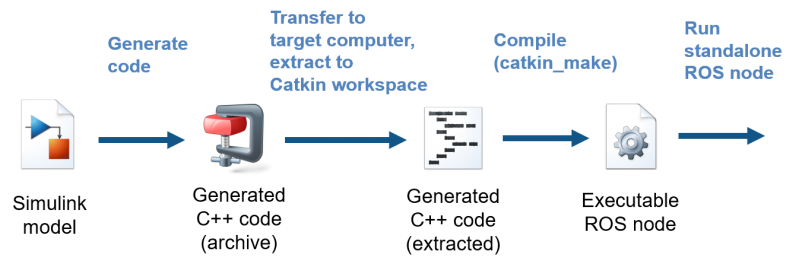# Working with a Generated Node

Once you’ve generated a ROS node from Simulink, there are a few ways you can work with it.

• Start and stop the node from MATLAB using rosdevice, or run it directly from your ROS machine by finding the generated executable or using the rosrun
• Use External mode to tune parameters and visualize data directly in the Simulink model, even though the code itself is running on the target computer. The code will be generated with a TCP/IP interface to your Simulink model running on the desktop. While this has some processing overhead, it can be a good debugging step while you are still designing your algorithm.
• Configure your algorithm to send or receive ROS messages or values in the parameter server. This lets you interact with the deployed node from MATLAB, Simulink, or other ROS nodes and the terminal on your target machine.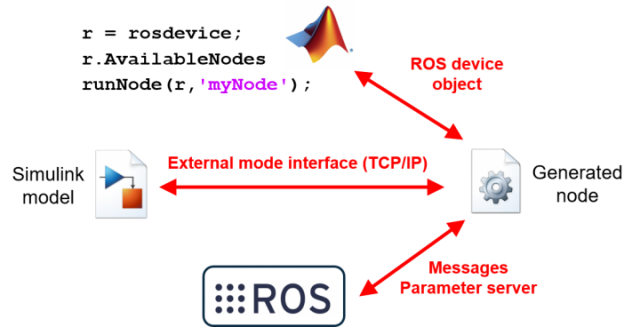For more information on deploying algorithms as standalone ROS nodes, and using the deployed nodes, watch the following videos.
MATLAB and Simulink Robotics Arena: Deploying Algorithms to ROS
MATLAB and Simulink Robotics Arena: Designing Distributed Systems with ROS

# Conclusion

You have seen an overview of how algorithms can be prototyped in MATLAB and Simulink, and how they can then become standalone C++ based ROS nodes. If you’d like to know more, leave us a comment or post on our Facebook group.

You can download the templates and examples shown in the videos from the MATLAB Central File Exchange. The virtual machine used in the examples can be downloaded from the MathWorks Web site.

– Sebastian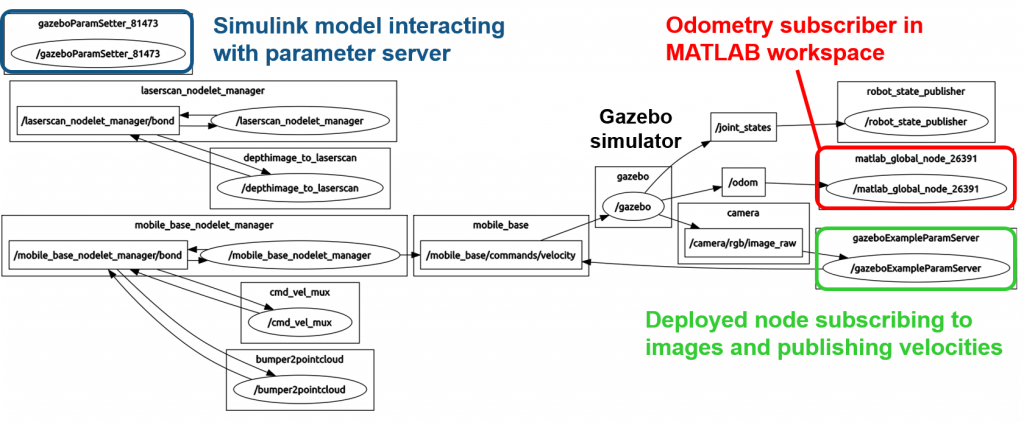An example of MATLAB and Simulink interfacing with ROS, as shown by the ROS utility rqt_graph.

|